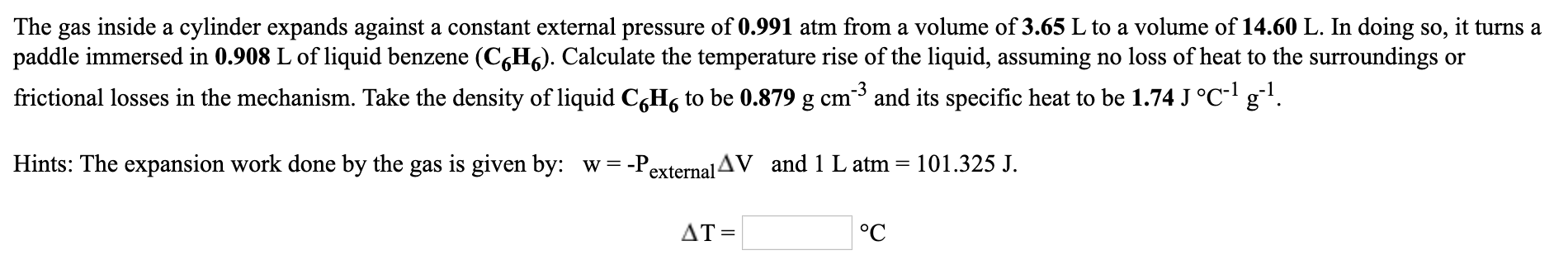# The gas inside a cylinder expands against a constant external pressure of 0.991 atm from a volume of 3.65 L to a volume of 14.60 L. In doing so, it turns apaddle immersed in 0.908 L of liquid benzene (C6H6). Calculate the temperature rise of the liquid, assuming no loss of heat to the surroundings orfrictional losses in the mechanism. Take the density of liquid CgH, to be 0.879 g cm3 and its specific heat to be 1.74 J °C- g.Hints: The expansion work done by the gas is given by: w = -PexternalAV and 1 L atm =101.325 J.AT =°C

Question
1 viewshelp_outlineImage TranscriptioncloseThe gas inside a cylinder expands against a constant external pressure of 0.991 atm from a volume of 3.65 L to a volume of 14.60 L. In doing so, it turns a paddle immersed in 0.908 L of liquid benzene (C6H6). Calculate the temperature rise of the liquid, assuming no loss of heat to the surroundings or frictional losses in the mechanism. Take the density of liquid CgH, to be 0.879 g cm3 and its specific heat to be 1.74 J °C- g. Hints: The expansion work done by the gas is given by: w = -P external AV and 1 L atm = 101.325 J. AT = °C fullscreen
check_circle

Step 1

Since, there is no heat loss. Hence all the work don...

### Want to see the full answer?

See Solution

#### Want to see this answer and more?

Solutions are written by subject experts who are available 24/7. Questions are typically answered within 1 hour.*

See Solution
*Response times may vary by subject and question.
Tagged in